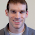## Monday, May 2, 2011

### Plotting the posterior probabilities on the tree (using pies!)

I thought I would post a few words on plotting the posterior probabilities of the rate shift on the tree. This follows specifically from my previous post here.

We can, of course, do this using edgelabels() from {ape} and plot the probabilities in numeric form (boring). We can also use pie graphs! This makes more sense here than for, say, plotting posterior clade probabilities from Bayesian phylogeny inference because in our MCMC method the rate shift must occur somewhere in the tree, so the posterior probabilities of it being anywhere have to sum to 1.0. (Consequently most of the posterior probabilities will often be close to zero and can be left off - which helps us avoid being overwhelms by pies!)

Picking up our simulated example, from before, we can plot the posterior probabilities (for all p>0.01) of the rate shifts on the tree using the following commands:

> p<-hist(mcmc[,"node"], breaks=0.5:(length(tree\$tip)+tree\$Nnode+0.5))
> plot(tree)
> edgelabels(edge=match(which(p\$density>0.01),tree\$edge[,2]), pie=p\$density[which(p\$density>0.01)],piecol=c("black","white"))

Which should produce something close to the tree below:Note, that we have simulated a very large effect on the evolutionary rate, which explains why the posterior density of the rate shift is so highly concentrated on the correct edge. For empirical data, the posterior density will often be more dispersed across the branches of the tree.

#### 1 comment:

1.The title of this post was not intended to be alliterative.

Note: due to the very large amount of spam, all comments are now automatically submitted for moderation.